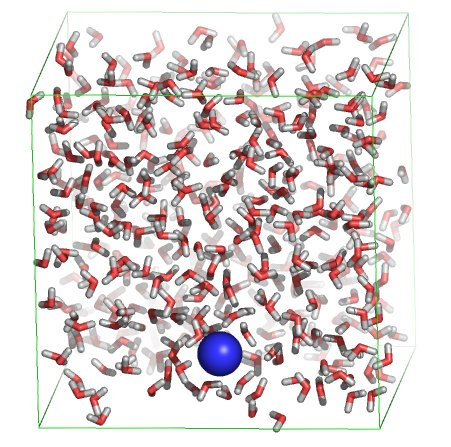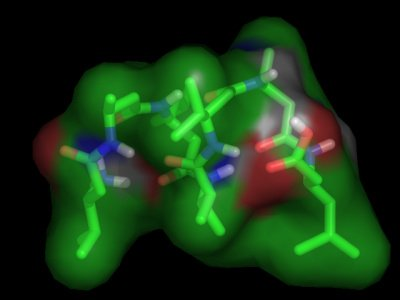# Free energy calculationsMolecular simulations can be carried out to gain either dynamical or

energetical information on the simulated system. Today we'll focus on

different ways to extract energies and free energies from simulations.

### Solvation free energy of a sodium ion in water

One way to gain access to free energies by simulation is to perform

so-called free-energy perturbation (FEP) simulations. In such

simulations, the system is gradually "morphed" from one state to

another (let's say from state "A" to state "B"), during which the

effect of this perturbation onto the free energy is monitored. In

practice, this is achieved by defining a morph parameter lambda, which

is defined to be zero in the initial state (A), and one in the final

state (B). By collecting the derivative of the potential energy with

respect to lambda during the simulation, and by integrating over

lambda afterwards, we gain access to the free energy. We are now going

to use this procedure to calculate the free energy of solvation for a

sodium ion in water. For this, we'll slowly create a sodium ion in a

topology, and the MD parameter file first.

First have a look at the starting structure:

In rasmol, type:

##### cpk

Note that the sodium ion is already "present" in the starting

structure. Now have a look at the topology "na.top", with 'xedit',

'nedit', 'more' or 'less', and look for the line containing "Na" under

"[atoms]". You'll notice that two states (A and B) are defined, with

charge 0 and 1, respectively. This means that during the simulation,

the charge of the sodium ion will be slowly switched on.

Now start the simulation:

##### mdrun -v -c charged.gro

Analyse the free energy change by:

##### xmgrace dgdl.xvg

In order to compute the free energy, we must integrate dG/dlambda over

dlambda. Instead, we have dG/dlambda as a function of time. This is

not a problem, as we know that lambda changed from0 to 1 during this

time (10 ps). So instead, we can integrate the curve over time, and

divide the obtained value by ten to derive the desired free energy.

To integrate, under "Data", select "Transformations", followed by

"Integration", and press "Accept".

#### Question:

The experimentally observed solvation free energies for sodium range

from -365 to -372 kJ/mol. How does the obtained value correspond to

that?

Instead of integrating dG/dlambda, we can also compare the initial and

final values of the potential energy of the system:

##### g_energy

Select "Potential", followed by "0" (and, if necessary, an extra "enter").

##### xmgrace energy.xvg

Subtract the initial value from the final value.

#### Question:

What value do you get? Why is this value so much larger (in absolute

value) than the free energy difference obtained by integrating

dG/dlambda?

One important check in simulations in general and in free energy

simulations in particular is to make sure that the answer has

converged. In order to do so, we can either perform a longer (or

shorter!) simulation, and compare the result to the original, or we

can perform the backward transition to see if the free energy

difference is the opposite of the forward transition. In this

practical, we'll do both. First, we'll carry out the backward

transition. Copy the MD parameter file to incorporate the changes:

##### cp fep.mdp back.mdp

and open "back.mdp" in your favorite editor (xedit, nedit, emacs,

kate, vi)

to make the following changes:

First search for "Free energy", then change "init-lambda" to 1 and

change delta-lambda to "-0.0002". Now we can start the backward

simulation. For this, we'll use the final structure of the previous

simulation (charged.gro) as initial structure:

##### mdrun -v

And analyse the free energy change using xmgrace. Remember that we now

changed lambda from one to zero, meaning that if we integrate over

time, we should divide the obtained value by -10 instead of by 10.

#### Question:

What value do you get? How does this value compare to the free energy

change for the forward simulation? Would you consider the obtained

value for the solvation free energy sufficiently converged?

#### Question:

If we take the forward and backward

simulation together, is the total free energy change positive or

negative? Which of the two should it have been?

As stated above, another way to check convergence is to perform a

longer simulation and see if the free energy change remains the same

as compared to a shorter simulation. For this, open "fep.mdp" in your

favorite editor. Change "nsteps" from 5000 to 50000, and

"delta-lambda" from 0.0002 to 0.00002, and repeat the forward

transition:

#### Question:

Is the obtained free energy very different as compared to the shorter

simulations? What would you consider an appropriate length for this

type of simulation?

### Thermodynamics of reversible peptide foldingFor the case of a sodium ion in water we could compute the free energy

difference between two states because we defined a path connecting the

path. In the case of the ion in water, the path was an artificial path

(hence such free energy perturbation studies are also called

"computational alchemy"). Likewise, a free energy difference can also

be computed along a real path, e.g. by forcing an ion across an

electrostatic gradient and integrating the force required to displace

the ion along the path.

There are also cases, however, where such a path, artificial or real,

can not be easily defined. Peptide or protein folding is a good

example. The problem of defining a path is that there are multiple

folding (and unfolding) pathways that connect the (well-defined)

folded state and the degenerate unfolded state. If we would like to

compute the folding free energy

by integrating the force along a pathway connecting the two, we'd

therefore have to follow multiple pathways, and take a weighted

average of the results. In the case, however, where we have a

simulation in which folding and unfolding takes place spontaneously

(i.e. without forcing the system to fold or unfold) and reversibly

we can also access the free energy difference between the folded and

unfolded state in a different way, namely simply by monitoring the

fraction of time the system resides in each state. In the equilibrium

case (i.e. if the simulation was long enough to visit all relevant

configurations multiple times) the probability of finding the system

is exactly proportional to its Boltzmann factor. Therefore, for any

two states, the following equation holds:

p1/p2 = exp (-DeltaG / kT)

with DeltaG = G1 - G2

Therefore, if we know the respective probability to find the system in

a particular pair of states, we can calculate the free energy

difference between these two states. Note that for this type of free

energy calculation we solely need the conformational information

(coordinates, trajectory) from the simulation, no energies!

To illustrate the above, we are going to calculate the free energy of

PDB file of the peptide and the MD trajectories at four different

temperatures: 298K, 340K,

350K and 360K.

First view part of one trajectory with pymol:

##### trjconv -s pep.pdb -f 340.xtc -o movie.pdb -e 10000 -fit rot+trans

and select "4" followed by "1" when asked to select a group.

in pymol, type:

##### show sticks

and press the "play" button on the bottom right of the main pymol

window. You see the first folding transition during the simulation at

340 K.

#### Question:

What kind of structure is the folded structure?

For a more quantitative analysis of the folding/unfolding

transitions, we'll analyse the root mean square deviation of each

structure in each trajectory with respect to the folded

structure. Here we will call the structure "folded" if it has a root

mean square deviation (RMSD) of less than 2 Angstroms (0.2 nm) of the

backbone atoms with respect to the reference structure in pep.pdb.

##### g_rms -s pep.pdb -f 340.xtc -o rmsd_340.xvg

when asked for a selection of groups, press "4" and "4" again.

View the result with xmgrace:

#### Question:

How many folding/unfolding transitions do you observe?

Repeat the same procedure for the other 3 temperatures to generate

rmsd_298.xvg, rmsd_350.xvg and rmsd_360.xvg. With a command like the

following, we can count how many configuration from each trajectory

are "folded" or "unfolded":

##### tail +11 rmsd_340.xvg | awk '$2 < 0.2 {print$0}' | wc -l

if the counting didn't work, then try:

##### tail +11 rmsd_340.xvg | sed 's/0\./0,/g' | awk '$2 < 0.2 {print$0}' | wc -l

(never mind if you didn't understand that command completely. The

"tail" part throws away the header of the rmsd_340.xvg file, the

output is then "piped" to the "awk" command that filters the output and

only prints the lines of which the second column (the RMSD) is smaller

than 0.2; the "wc -l" simply counts the number of lines). The sed command,

finally, replaces the dots in the file with comma's, for the case your

computer has expects german input, which uses comma's for floating point numbers.

The obtained number is the number of "folded" configurations in the 340K

simulation. Repeat the procedure, now to count the number of

"unfolded" conformations. You'll see, at this temperature the system

is folded about one third of the time.

#### Question:

What is the difference in free energy between the folded and unfolded

state at this temperature? (Hint: kT = 2.5 kJ/mol at 300K)

#### Question:

What about the other three temperatures? What would you estimate to be

the folding temperature?

#### Question:

Assuming that the folding enthalpy is the same for the different

temperatures, how can the entropy difference between the folded and

unfolded state be estimated from the set of free energies? How large

do you estimate the entropy of folding in this case? Is it positive or

negative? Why?

Go to Editor View